## 3.2  The Environment Model of Evaluation

When we introduced compound procedures in chapter 1, we used the substitution model of evaluation (section 1.1.5) to define what is meant by applying a procedure to arguments:

• To apply a compound procedure to arguments, evaluate the body of the procedure with each formal parameter replaced by the corresponding argument.

Once we admit assignment into our programming language, such a definition is no longer adequate. In particular, section 3.1.3 argued that, in the presence of assignment, a variable can no longer be considered to be merely a name for a value. Rather, a variable must somehow designate a ``place'' in which values can be stored. In our new model of evaluation, these places will be maintained in structures called environments.

An environment is a sequence of frames. Each frame is a table (possibly empty) of bindings, which associate variable names with their corresponding values. (A single frame may contain at most one binding for any variable.) Each frame also has a pointer to its enclosing environment, unless, for the purposes of discussion, the frame is considered to be global. The value of a variable with respect to an environment is the value given by the binding of the variable in the first frame in the environment that contains a binding for that variable. If no frame in the sequence specifies a binding for the variable, then the variable is said to be unbound in the environment.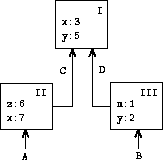Figure 3.1 shows a simple environment structure consisting of three frames, labeled I, II, and III. In the diagram, A, B, C, and D are pointers to environments. C and D point to the same environment. The variables z and x are bound in frame II, while y and x are bound in frame I. The value of x in environment D is 3. The value of x with respect to environment B is also 3. This is determined as follows: We examine the first frame in the sequence (frame III) and do not find a binding for x, so we proceed to the enclosing environment D and find the binding in frame I. On the other hand, the value of x in environment A is 7, because the first frame in the sequence (frame II) contains a binding of x to 7. With respect to environment A, the binding of x to 7 in frame II is said to shadow the binding of x to 3 in frame I.

The environment is crucial to the evaluation process, because it determines the context in which an expression should be evaluated. Indeed, one could say that expressions in a programming language do not, in themselves, have any meaning. Rather, an expression acquires a meaning only with respect to some environment in which it is evaluated. Even the interpretation of an expression as straightforward as (+ 1 1) depends on an understanding that one is operating in a context in which + is the symbol for addition. Thus, in our model of evaluation we will always speak of evaluating an expression with respect to some environment. To describe interactions with the interpreter, we will suppose that there is a global environment, consisting of a single frame (with no enclosing environment) that includes values for the symbols associated with the primitive procedures. For example, the idea that + is the symbol for addition is captured by saying that the symbol + is bound in the global environment to the primitive addition procedure.

### 3.2.1  The Rules for Evaluation

The overall specification of how the interpreter evaluates a combination remains the same as when we first introduced it in section 1.1.3:

• To evaluate a combination:

1.  Evaluate the subexpressions of the combination.12

2.  Apply the value of the operator subexpression to the values of the operand subexpressions.

The environment model of evaluation replaces the substitution model in specifying what it means to apply a compound procedure to arguments.

In the environment model of evaluation, a procedure is always a pair consisting of some code and a pointer to an environment. Procedures are created in one way only: by evaluating a lambda expression. This produces a procedure whose code is obtained from the text of the lambda expression and whose environment is the environment in which the lambda expression was evaluated to produce the procedure. For example, consider the procedure definition

(define (square x)
(* x x))

evaluated in the global environment. The procedure definition syntax is just syntactic sugar for an underlying implicit lambda expression. It would have been equivalent to have used

(define square
(lambda (x) (* x x)))

which evaluates (lambda (x) (* x x)) and binds square to the resulting value, all in the global environment.

Figure 3.2 shows the result of evaluating this define expression. The procedure object is a pair whose code specifies that the procedure has one formal parameter, namely x, and a procedure body (* x x). The environment part of the procedure is a pointer to the global environment, since that is the environment in which the lambda expression was evaluated to produce the procedure. A new binding, which associates the procedure object with the symbol square, has been added to the global frame. In general, define creates definitions by adding bindings to frames.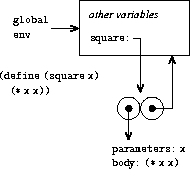Now that we have seen how procedures are created, we can describe how procedures are applied. The environment model specifies: To apply a procedure to arguments, create a new environment containing a frame that binds the parameters to the values of the arguments. The enclosing environment of this frame is the environment specified by the procedure. Now, within this new environment, evaluate the procedure body.

To show how this rule is followed, figure 3.3 illustrates the environment structure created by evaluating the expression (square 5) in the global environment, where square is the procedure generated in figure 3.2. Applying the procedure results in the creation of a new environment, labeled E1 in the figure, that begins with a frame in which x, the formal parameter for the procedure, is bound to the argument 5. The pointer leading upward from this frame shows that the frame's enclosing environment is the global environment. The global environment is chosen here, because this is the environment that is indicated as part of the square procedure object. Within E1, we evaluate the body of the procedure, (* x x). Since the value of x in E1 is 5, the result is (* 5 5), or 25.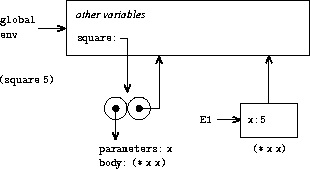The environment model of procedure application can be summarized by two rules:

• A procedure object is applied to a set of arguments by constructing a frame, binding the formal parameters of the procedure to the arguments of the call, and then evaluating the body of the procedure in the context of the new environment constructed. The new frame has as its enclosing environment the environment part of the procedure object being applied.

• A procedure is created by evaluating a lambda expression relative to a given environment. The resulting procedure object is a pair consisting of the text of the lambda expression and a pointer to the environment in which the procedure was created.

We also specify that defining a symbol using define creates a binding in the current environment frame and assigns to the symbol the indicated value.13 Finally, we specify the behavior of set!, the operation that forced us to introduce the environment model in the first place. Evaluating the expression (set! <variable> <value>) in some environment locates the binding of the variable in the environment and changes that binding to indicate the new value. That is, one finds the first frame in the environment that contains a binding for the variable and modifies that frame. If the variable is unbound in the environment, then set! signals an error.

These evaluation rules, though considerably more complex than the substitution model, are still reasonably straightforward. Moreover, the evaluation model, though abstract, provides a correct description of how the interpreter evaluates expressions. In chapter 4 we shall see how this model can serve as a blueprint for implementing a working interpreter. The following sections elaborate the details of the model by analyzing some illustrative programs.

### 3.2.2  Applying Simple Procedures

When we introduced the substitution model in section 1.1.5 we showed how the combination (f 5) evaluates to 136, given the following procedure definitions:

(define (square x)
(* x x))
(define (sum-of-squares x y)
(+ (square x) (square y)))
(define (f a)
(sum-of-squares (+ a 1) (* a 2)))

We can analyze the same example using the environment model. Figure 3.4 shows the three procedure objects created by evaluating the definitions of f, square, and sum-of-squares in the global environment. Each procedure object consists of some code, together with a pointer to the global environment.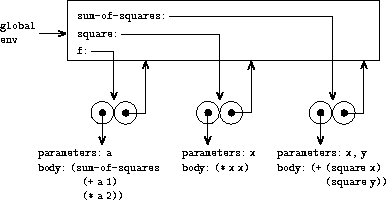In figure 3.5 we see the environment structure created by evaluating the expression (f 5). The call to f creates a new environment E1 beginning with a frame in which a, the formal parameter of f, is bound to the argument 5. In E1, we evaluate the body of f:

(sum-of-squares (+ a 1) (* a 2))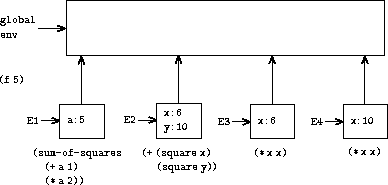To evaluate this combination, we first evaluate the subexpressions. The first subexpression, sum-of-squares, has a value that is a procedure object. (Notice how this value is found: We first look in the first frame of E1, which contains no binding for sum-of-squares. Then we proceed to the enclosing environment, i.e. the global environment, and find the binding shown in figure 3.4.) The other two subexpressions are evaluated by applying the primitive operations + and * to evaluate the two combinations (+ a 1) and (* a 2) to obtain 6 and 10, respectively.

Now we apply the procedure object sum-of-squares to the arguments 6 and 10. This results in a new environment E2 in which the formal parameters x and y are bound to the arguments. Within E2 we evaluate the combination (+ (square x) (square y)). This leads us to evaluate (square x), where square is found in the global frame and x is 6. Once again, we set up a new environment, E3, in which x is bound to 6, and within this we evaluate the body of square, which is (* x x). Also as part of applying sum-of-squares, we must evaluate the subexpression (square y), where y is 10. This second call to square creates another environment, E4, in which x, the formal parameter of square, is bound to 10. And within E4 we must evaluate (* x x).

The important point to observe is that each call to square creates a new environment containing a binding for x. We can see here how the different frames serve to keep separate the different local variables all named x. Notice that each frame created by square points to the global environment, since this is the environment indicated by the square procedure object.

After the subexpressions are evaluated, the results are returned. The values generated by the two calls to square are added by sum-of-squares, and this result is returned by f. Since our focus here is on the environment structures, we will not dwell on how these returned values are passed from call to call; however, this is also an important aspect of the evaluation process, and we will return to it in detail in chapter 5.

Exercise 3.9.  In section 1.2.1 we used the substitution model to analyze two procedures for computing factorials, a recursive version

(define (factorial n)
(if (= n 1)
1
(* n (factorial (- n 1)))))

and an iterative version

(define (factorial n)
(fact-iter 1 1 n))
(define (fact-iter product counter max-count)
(if (> counter max-count)
product
(fact-iter (* counter product)
(+ counter 1)
max-count)))

Show the environment structures created by evaluating (factorial 6) using each version of the factorial procedure.14

### 3.2.3  Frames as the Repository of Local State

We can turn to the environment model to see how procedures and assignment can be used to represent objects with local state. As an example, consider the ``withdrawal processor'' from section 3.1.1 created by calling the procedure

(define (make-withdraw balance)
(lambda (amount)
(if (>= balance amount)
(begin (set! balance (- balance amount))
balance)
"Insufficient funds")))

Let us describe the evaluation of

(define W1 (make-withdraw 100))

followed by

(W1 50)
50

Figure 3.6 shows the result of defining the make-withdraw procedure in the global environment. This produces a procedure object that contains a pointer to the global environment. So far, this is no different from the examples we have already seen, except that the body of the procedure is itself a lambda expression.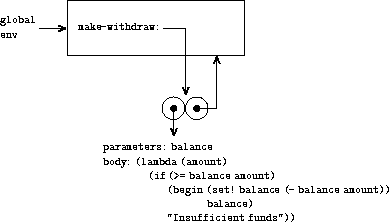The interesting part of the computation happens when we apply the procedure make-withdraw to an argument:

(define W1 (make-withdraw 100))

We begin, as usual, by setting up an environment E1 in which the formal parameter balance is bound to the argument 100. Within this environment, we evaluate the body of make-withdraw, namely the lambda expression. This constructs a new procedure object, whose code is as specified by the lambda and whose environment is E1, the environment in which the lambda was evaluated to produce the procedure. The resulting procedure object is the value returned by the call to make-withdraw. This is bound to W1 in the global environment, since the define itself is being evaluated in the global environment. Figure 3.7 shows the resulting environment structure.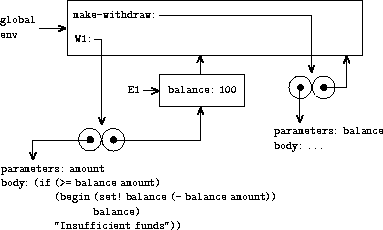Now we can analyze what happens when W1 is applied to an argument:

(W1 50)
50

We begin by constructing a frame in which amount, the formal parameter of W1, is bound to the argument 50. The crucial point to observe is that this frame has as its enclosing environment not the global environment, but rather the environment E1, because this is the environment that is specified by the W1 procedure object. Within this new environment, we evaluate the body of the procedure:

(if (>= balance amount)
(begin (set! balance (- balance amount))
balance)
"Insufficient funds")

The resulting environment structure is shown in figure 3.8. The expression being evaluated references both amount and balance. Amount will be found in the first frame in the environment, while balance will be found by following the enclosing-environment pointer to E1.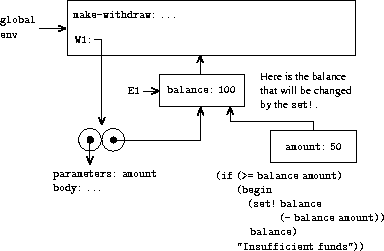When the set! is executed, the binding of balance in E1 is changed. At the completion of the call to W1, balance is 50, and the frame that contains balance is still pointed to by the procedure object W1. The frame that binds amount (in which we executed the code that changed balance) is no longer relevant, since the procedure call that constructed it has terminated, and there are no pointers to that frame from other parts of the environment. The next time W1 is called, this will build a new frame that binds amount and whose enclosing environment is E1. We see that E1 serves as the ``place'' that holds the local state variable for the procedure object W1. Figure 3.9 shows the situation after the call to W1.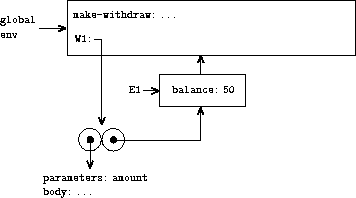Observe what happens when we create a second ``withdraw'' object by making another call to make-withdraw:

(define W2 (make-withdraw 100))

This produces the environment structure of figure 3.10, which shows that W2 is a procedure object, that is, a pair with some code and an environment. The environment E2 for W2 was created by the call to make-withdraw. It contains a frame with its own local binding for balance. On the other hand, W1 and W2 have the same code: the code specified by the lambda expression in the body of make-withdraw.15 We see here why W1 and W2 behave as independent objects. Calls to W1 reference the state variable balance stored in E1, whereas calls to W2 reference the balance stored in E2. Thus, changes to the local state of one object do not affect the other object.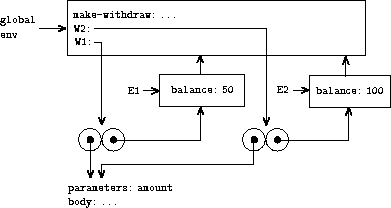Exercise 3.10.  In the make-withdraw procedure, the local variable balance is created as a parameter of make-withdraw. We could also create the local state variable explicitly, using let, as follows:

(define (make-withdraw initial-amount)
(let ((balance initial-amount))
(lambda (amount)
(if (>= balance amount)
(begin (set! balance (- balance amount))
balance)
"Insufficient funds"))))

Recall from section 1.3.2 that let is simply syntactic sugar for a procedure call:

(let ((<var> <exp>)) <body>)

is interpreted as an alternate syntax for

((lambda (<var>) <body>) <exp>)

Use the environment model to analyze this alternate version of make-withdraw, drawing figures like the ones above to illustrate the interactions

(define W1 (make-withdraw 100))

(W1 50)

(define W2 (make-withdraw 100))

Show that the two versions of make-withdraw create objects with the same behavior. How do the environment structures differ for the two versions?

### 3.2.4  Internal Definitions

Section 1.1.8 introduced the idea that procedures can have internal definitions, thus leading to a block structure as in the following procedure to compute square roots:

(define (sqrt x)
(define (good-enough? guess)
(< (abs (- (square guess) x)) 0.001))
(define (improve guess)
(average guess (/ x guess)))
(define (sqrt-iter guess)
(if (good-enough? guess)
guess
(sqrt-iter (improve guess))))
(sqrt-iter 1.0))

Now we can use the environment model to see why these internal definitions behave as desired. Figure 3.11 shows the point in the evaluation of the expression (sqrt 2) where the internal procedure good-enough? has been called for the first time with guess equal to 1.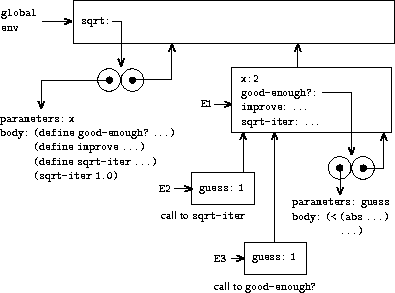Observe the structure of the environment. Sqrt is a symbol in the global environment that is bound to a procedure object whose associated environment is the global environment. When sqrt was called, a new environment E1 was formed, subordinate to the global environment, in which the parameter x is bound to 2. The body of sqrt was then evaluated in E1. Since the first expression in the body of sqrt is

(define (good-enough? guess)
(< (abs (- (square guess) x)) 0.001))

evaluating this expression defined the procedure good-enough? in the environment E1. To be more precise, the symbol good-enough? was added to the first frame of E1, bound to a procedure object whose associated environment is E1. Similarly, improve and sqrt-iter were defined as procedures in E1. For conciseness, figure 3.11 shows only the procedure object for good-enough?.

After the local procedures were defined, the expression (sqrt-iter 1.0) was evaluated, still in environment E1. So the procedure object bound to sqrt-iter in E1 was called with 1 as an argument. This created an environment E2 in which guess, the parameter of sqrt-iter, is bound to 1. Sqrt-iter in turn called good-enough? with the value of guess (from E2) as the argument for good-enough?. This set up another environment, E3, in which guess (the parameter of good-enough?) is bound to 1. Although sqrt-iter and good-enough? both have a parameter named guess, these are two distinct local variables located in different frames. Also, E2 and E3 both have E1 as their enclosing environment, because the sqrt-iter and good-enough? procedures both have E1 as their environment part. One consequence of this is that the symbol x that appears in the body of good-enough? will reference the binding of x that appears in E1, namely the value of x with which the original sqrt procedure was called. The environment model thus explains the two key properties that make local procedure definitions a useful technique for modularizing programs:

• The names of the local procedures do not interfere with names external to the enclosing procedure, because the local procedure names will be bound in the frame that the procedure creates when it is run, rather than being bound in the global environment.

• The local procedures can access the arguments of the enclosing procedure, simply by using parameter names as free variables. This is because the body of the local procedure is evaluated in an environment that is subordinate to the evaluation environment for the enclosing procedure.

Exercise 3.11.  In section 3.2.3 we saw how the environment model described the behavior of procedures with local state. Now we have seen how internal definitions work. A typical message-passing procedure contains both of these aspects. Consider the bank account procedure of section 3.1.1:

(define (make-account balance)
(define (withdraw amount)
(if (>= balance amount)
(begin (set! balance (- balance amount))
balance)
"Insufficient funds"))
(define (deposit amount)
(set! balance (+ balance amount))
balance)
(define (dispatch m)
(cond ((eq? m 'withdraw) withdraw)
((eq? m 'deposit) deposit)
(else (error "Unknown request -- MAKE-ACCOUNT"
m))))
dispatch)

Show the environment structure generated by the sequence of interactions

(define acc (make-account 50))

((acc 'deposit) 40)
90

((acc 'withdraw) 60)
30

Where is the local state for acc kept? Suppose we define another account

(define acc2 (make-account 100))

How are the local states for the two accounts kept distinct? Which parts of the environment structure are shared between acc and acc2?

12 Assignment introduces a subtlety into step 1 of the evaluation rule. As shown in exercise 3.8, the presence of assignment allows us to write expressions that will produce different values depending on the order in which the subexpressions in a combination are evaluated. Thus, to be precise, we should specify an evaluation order in step 1 (e.g., left to right or right to left). However, this order should always be considered to be an implementation detail, and one should never write programs that depend on some particular order. For instance, a sophisticated compiler might optimize a program by varying the order in which subexpressions are evaluated.

13 If there is already a binding for the variable in the current frame, then the binding is changed. This is convenient because it allows redefinition of symbols; however, it also means that define can be used to change values, and this brings up the issues of assignment without explicitly using set!. Because of this, some people prefer redefinitions of existing symbols to signal errors or warnings.

14 The environment model will not clarify our claim in section 1.2.1 that the interpreter can execute a procedure such as fact-iter in a constant amount of space using tail recursion. We will discuss tail recursion when we deal with the control structure of the interpreter in section 5.4.

15 Whether W1 and W2 share the same physical code stored in the computer, or whether they each keep a copy of the code, is a detail of the implementation. For the interpreter we implement in chapter 4, the code is in fact shared.